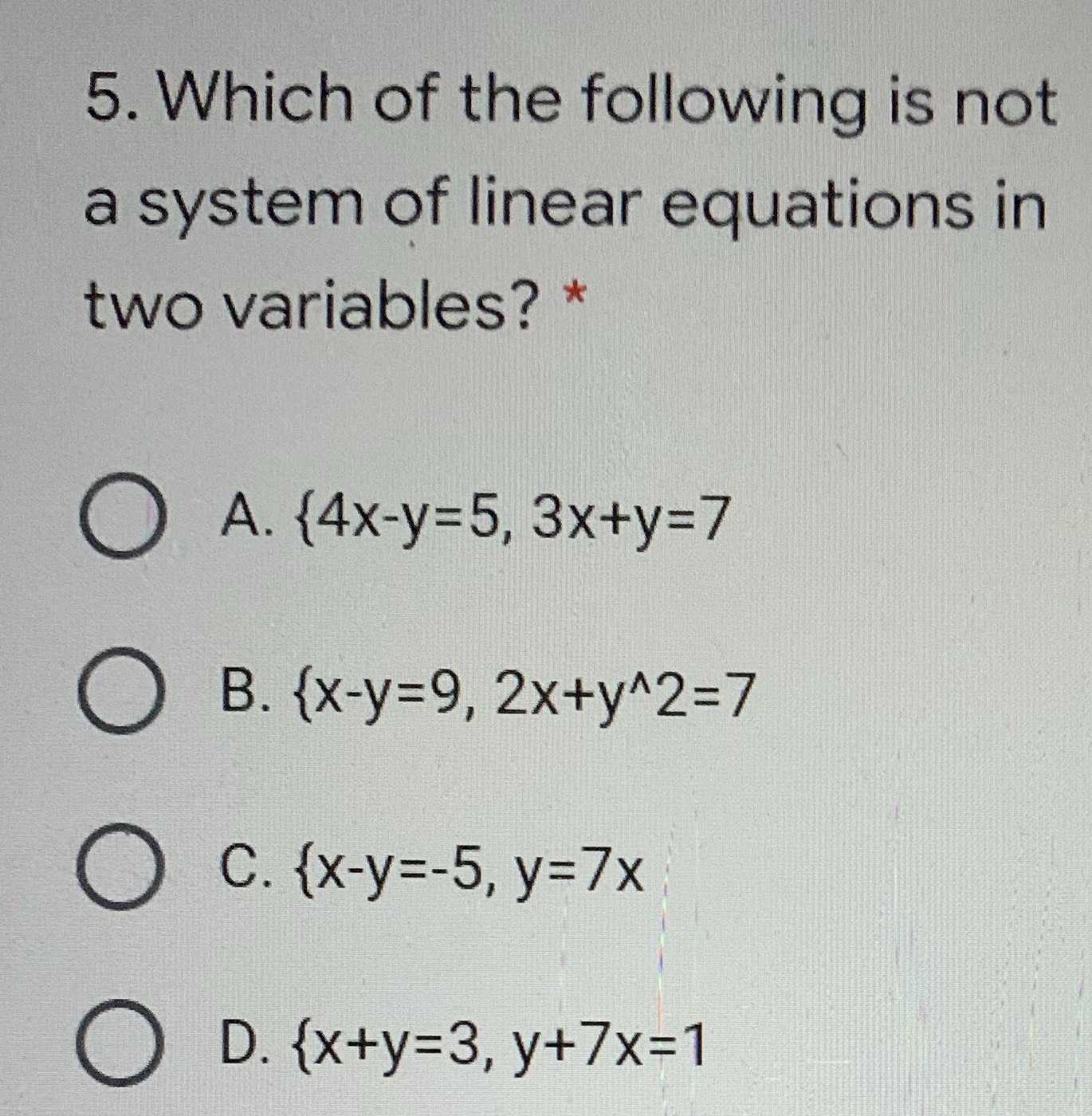### ¿Todavía tienes preguntas de matemáticas?

Pregunte a nuestros tutores expertos
Algebra
Pregunta5. Which of the following is not a system of linear equations in two variables? *

A. $$\{ 4 x - y = 5,3 x + y = 7$$

B. $$\{ x - y = 9,2 x + y ^ { \wedge } 2 = 7$$

C. $$\{ x - y = - 5 , y = 7 x$$

D. $$\{ x + y = 3 , y + 7 x = 1$$